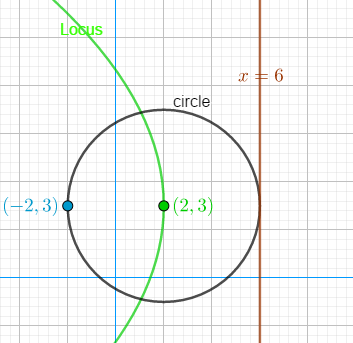### Math Notes

Subjects

#### Analytic Geometry Solutions

##### Topics || Problems

Find the locus of the center of a circle which passes through (-2,3) and touches the line x=6.

A circle is moving in which at one instance the circle touches the point $$(-2,3)$$ and tangent to the line $$x=6$$.

To calculate the locus of the center of the circle, equate the distance between the center of the circle to the distance (perpendicular) between the center of the circle and the line.

Let $$(x,y)$$ be the center of the circle. The distance between the center of the circle to the point $$(-2,3)$$ is $$d^2 =(x+2)^2+ (y-3)^2$$ and the distance between the center of the circle to the line is $$d = \frac{1(x) + 0(y) - 6 }{\sqrt{1^2+0}} = x - 6$$

$$(x+2)^2 + (y-3)^2 = (x-6)^2$$

$$x^2 +4x+4+ y^2 -6y +9 = x^2 -12x +36$$

$$y^2 -6y + 16x-23 =0$$ AnswerDistance between two points $$(x,y) ~ and ~(x,_1,y_1)$$: $$d^2 = (x_1-x)^2+(y_1-y)^2$$

Distance between a point $$(x_o, y_o)$$ and a line $$(Ax + By + C = 0)$$: $$d = \frac{Ax_o + By_o +C}{\sqrt{A^2+B^2}}$$